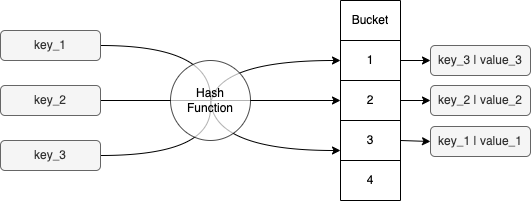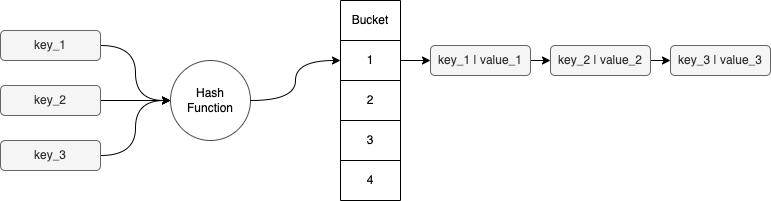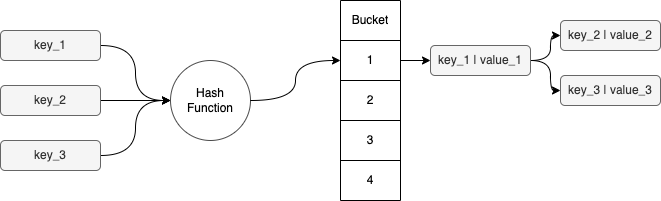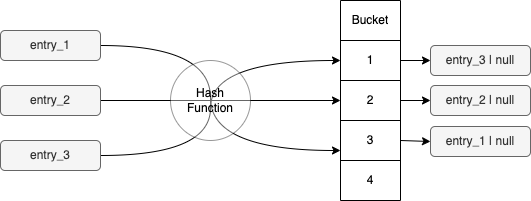# Yonatan Karp-Rudin# How does HashMap work in Java?

## Delving Deep into Java HashMaps: A Comprehensive Guide

As an interviewer, I often ask the question about how HashMap works in Java. It helps me assess a candidate's understanding of data structures, JVM internals, and their problem-solving approach. I've encountered many candidates, including juniors, mid-levels, and seniors, who couldn't answer this question correctly. So, I decided to share the answer with everyone.

In this article, I will explain the HashMap question step by step.

### What is the contract between the equals() and hashCode() functions in Java?In Java, all objects inherit the `equals()` and `hashCode()` functions from the `Object` class. According to the documentation of `equals()`, it's necessary to override the `hashCode()` method as well. This ensures that equal objects have equal hash codes.

If we read the documentation of `equals()`, we can notice the following:

Note that it is generally necessary to override the `hashCode` method whenever this method is overridden, so as to maintain the general contract for the `hashCode` method, which states that equal objects must have equal hash codes.

But what does that mean?

It means that if we have two objects considered equal, they must have the same hash code. However, if the objects are not equal, there is no guarantee that they would have different hash codes.

### What is the complexity of retrieving an element from a HashMap?Retrieving an element from a HashMap has a constant time complexity of `O(1)`.

That was easy! Or is it?

### What happens if hashCode() is overridden to return a constant value?

Let’s assume that as a developer, I have created the following class:

``````class MyClass {
@Override
public boolean equals(Object other) {
if (!(other instanceof MyClass)) return false;
return this == other;
}

@Override
public int hashCode() {
return 1;
}
}
``````

And now, in my code, I am creating a million objects and storing them in a HashMap:

``````Map<MyClass, Integer> map = new HashMap<>();
IntStream.range(0, 1_000_000)
.forEach(i -> map.put(new MyClass(), i));
``````

How would the new `hashCode()` function impact our performance, if at all?

To answer this question, we must first dig into how exactly HashMap is working in Java.

When we add a new element to our map, the hash code of this key is calculated first. According to its value, a bucket is selected. That bucket will contain the value (in this case, our object). In case of collision (2 or more objects with the same hash code), the key and the value are stored as a pair using some sort of data structure (e.g., a linked list).Now, let’s assume that we want to get an element from the map. The following actions will happen:

1. The hash code of the key is calculated using the `hashCode()` function.

2. The HashMap iterates over the linked list using the `equals()` function. It will finish when it finds the correct pair of keys and values.

3. Once the correct key is found, the value is returned or null if no key was found.

Note that, in general, the assumption is that the hash function is evenly distributed. That means we expect a very small amount of elements in each bucket. Thus, the search for the correct key can be considered as a constant time.

Yet, in our example, all the keys are going to have the same hash code and, thus, end up in the same bucket. Hence, getting a value from the map will need (in the worst case) scanning the entire linked list. That would take a linear time of `O(n)`.### Can we improve the search time?

Our problem now is that the bucket uses a linked list, a data structure that is unsorted. What if we would use a data structure that is sorted by nature?

We can replace our bucket implementation with a balanced binary tree. By doing so, we can ensure that each element in the bucket could be found with the worst-case complexity of `O(log(n))`. This is much better than what we had before.In fact, since Java 8, the internal implementation of HashMap was changed exactly like that.

### Bonus: Implementing HashSet using HashMapA HashSet is a set (an unordered collection of elements), where the same hash code only exists once.We can easily implement a `HashSet` using `HashMap`. We will do so by using our value for the set as the key for the map and null as the value. In fact, that’s again exactly the implementation Java chose.

### Conclusion

As you can see, the question covers many areas:

• General understanding of data structures

• Understanding of Java internals

• Checking how the candidate thinks in case they do not remember the internals of Java (to be clear, nobody expects the Wikipedia answer to this question)

Moreover, the question could be extended to cover more topics such as concurrency, for example (how would you implement distributed HashMap?).